# Functor

A mapping from one category into another that is compatible with the category structure. More precisely, a covariant functor from a category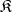into a category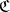or, simply, a functor frominto, is a pair of mappings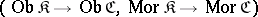, usually denoted by the same letter, for example(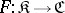), subject to the conditions:

1)for every;

2)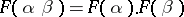for all morphisms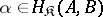,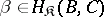.

A functor from the category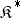dual tointo the categoryis called a contravariant functor frominto. Thus, for a contravariant functor, condition 1) must be satisfied as before, and condition 2) is replaced by: 2*)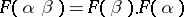for all morphisms,.

An-place functor from categories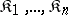intothat is covariant in the arguments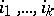and contravariant in the remaining arguments is a functor from the Cartesian productinto, where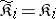for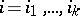and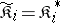for the remaining. Two-place functors that are covariant in both arguments are called bifunctors.

## Contents

### Examples of functors.

1) The identity mapping of a categoryonto itself is a covariant functor, called the identity functor of the category and denoted by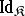or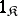.

2) Letbe an arbitrary locally small category, let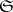be the category of sets, and letbe a fixed object of. If one associates to each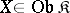the set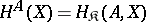and to each morphism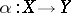the mapping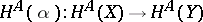, where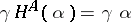for each, one obtains a functor frominto. This functor is called the covariant representable functor fromintowith representing object. Similarly, if one associates to an objectthe set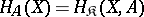and to a morphism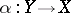the mapping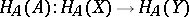, where, one obtains the contravariant representable functor fromintowith representing object. These functors are denoted byand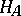, respectively. Ifis the category of vector spaces over a field, thentakes a spaceto its dual space of linear functionals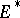. In the category of topological Abelian groups, the functor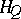, whereis the quotient group of the real numbers by the integers, associates to each group its group of characters.

3) If one associates to each pair of objectsandof an arbitrary category the set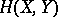, and to each pair of morphisms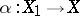and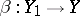the mapping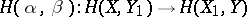defined by the equation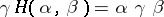for any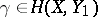, one obtains a two-place functor into the categorythat is contravariant in the first argument and covariant in the second.

In any category with finite products, the product can be regarded as an-place functor that is covariant in all arguments, for any natural number. As a rule, a construction that may be defined for any object of a category or for any sequence of objects of a fixed length, independently of the individual properties of the objects, is likely to be functorial. Examples of this are the construction of free algebras in some variety of universal algebras, which can be uniquely associated to each object of the category of sets; the construction of the fundamental group of a topological space, the construction of homology and cohomology groups of various dimensions; etc.

Any functordefines a mapping of each setinto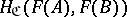which associates to a morphism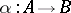the morphism. The functoris called faithful if these mappings are all injective, and full if they are all surjective. For every small category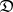, the assignment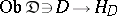can be extended to a full faithful functorfrominto the category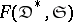of diagrams (cf. Diagram) with schemeover the category of sets.